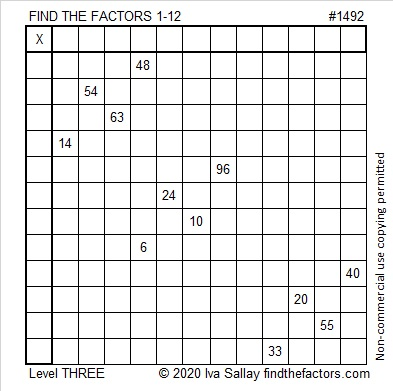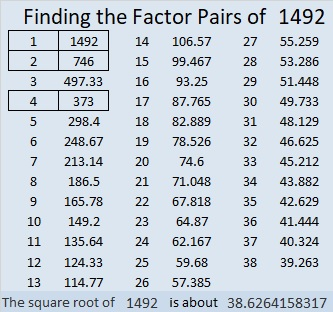# 1492 The Niña, the Pinta, and the Santa Maria

Contents

### Today’s Puzzle:

I debated with myself about whether or not I should mention the famous poem about 1492 in this post. Then I looked at the puzzle I had already created for today and noticed it would not be too much of a stretch to say the twelve clues look a little bit like three ships.  So whether or not I should, I decided to go ahead and mention the Niña, the Pinta, and the larger Santa Maria in the title. The ships themselves are innocent of any barbaric acts and might just be the three most famous ships in world history.

Start at the top of this level 3 puzzle and work your way down cell by cell using logic until you have written all the factors from 1 to 12 in both the first column and the top row.Here’s the same puzzle without color:### Factors of 1492:

• 1492 is a composite number.
• Prime factorization: 1492 = 2 × 2 × 373, which can be written 1492 = 2² × 373
• 1492 has at least one exponent greater than 1 in its prime factorization so √1492 can be simplified. Taking the factor pair from the factor pair table below with the largest square number factor, we get √1492 = (√4)(√373) = 2√373
• The exponents in the prime factorization are 2 and 1. Adding one to each exponent and multiplying we get (2 + 1)(1 + 1) = 3 × 2 = 6. Therefore 1492 has exactly 6 factors.
• The factors of 1492 are outlined with their factor pair partners in the graphic below.### Other Facts About the Number 1492:

1492 is the sum of two squares:
36² + 14² = 1492

1492 is the hypotenuse of a Pythagorean triple:
1008-1100-1492, which is 4 times (252-275-373)
and can also be calculated from 2(36)(14), 36² – 14², 36² + 14².

This site uses Akismet to reduce spam. Learn how your comment data is processed.